# 【优化求解】基于matlab差分进化算法求解函数极值问题【含Matlab源码 1199期】

## 一、简介

1 前言

2 差分进化算法理论 2.1差分进化算法原理 差分进化算法是一种随机的启发式搜索算法，简单易用，有较强的鲁棒性和全局寻优能力。它从数学角度看是一种随机搜索算法，从工程角度看是一种自适应的迭代寻优过程。除了具有较好的收敛性外，差分进化算法非常易于理解与执行，它只包含不多的几个控制参数，并且在整个迭代过程中，这些参数的值可以保持不变。差分进化算法是一种自组织最小化方法，用户只需很少的输入。它的关键思想与传统进化方法不同：传统方法是用预先确定的概率分布函数决定向量扰动；而差分进化算法的自组织程序利用种群中两个随机选择的不同向量来干扰一个现有向量，种群中的每一个向量都要进行干扰。差分进化算法利用一个向量种群，其中种群向量的随机扰动可独立进行，因此是并行的。如果新向量对应函数值的代价比它们的前辈代价小，它们将取代前辈向量。 同其他进化算法一样，差分进化算法也是对候选解的种群进行操作，但其种群繁殖方案与其他进化算法不同：它通过把种群中两个成员之间的加权差向量加到第三个成员上来产生新的参数向量，该操作 称为“变异”； 然后将变异向量的参数与另外预先确定的目标向量参数按一定规则混合来产生试验量，该操作称为“交叉”；最后，若试验向量的代价函数比目标向量的代价函数低，试验向量就在下一代中代替目标向量，该操作称为“选择”。种群中所有成员必须当作目标向量进行一次这样的操作，以便在下一代中出现相同个数竞争者。 在进化过程中对每一代的最佳参数向量都进行评价，以记录最小化过程。这样利用随机偏差扰动产生新个体的方式，可以获得一个收敛性非常好的结果，引导搜索过程向全局最优解逼近[6-7]。

2.2差分进化算法的特点 差分进化算法从提出到现在，在短短二十几年内人们对其进行了广泛的研究并取得了成功的应用。该算法主要有如下特点： (1)结构简单，容易使用。差分进化算法主要通过差分变异算子来进行遗传操作，由于该算子只涉及向量的加减运算，因此很容易实现；该算法采用概率转移规则，不需要确定性的规则。此外，差分进化算法的控制参数少，这些参数对算法性能的影响已经得到一定的研究，并得出了一些指导性的建议，因而可以方便使用人员根据问题选择较优的参数设置。 (2)性能优越。差分进化算法具有较好的可靠性、高效性和鲁棒性，对于大空间、非线性和不可求导的连续问题，其求解效率比其他进化方法好，而且很多学者还在对差分进化算法继续改良，以不断提高其性能。 (3)自适应性。差分进化算法的差分变异算子可以是固定常数，也可以具有变异步长和搜索方向自适应的能力，根据不同目标函数进行自动调整，从而提高搜索质量。 (4)差分进化算法具有内在的并行性，可协同搜索，具有利用个体局部信息和群体全局信息指导算法进一步搜索的能力。在同样精度要求下，差分进化算法具有更快的收敛速度。 (5)算法通用，可直接对结构对象进行操作，不依赖于问题信息，不存在对目标函数的限定。差分进化算法操作十分简单，易于编程实现，尤其利于求解高维的函数优化问题。

3 差分进化算法种类 3.1基本差分进化算法 基本差分进化算法的操作程序如下： (1)初始化； (2)变异； (3)交叉； (4)选择； (5)边界条件处理。 初始化 差分进化算法利用NP个维数为D的实数值参数向量，将它们作为每 一代的种群，每个个体表示为：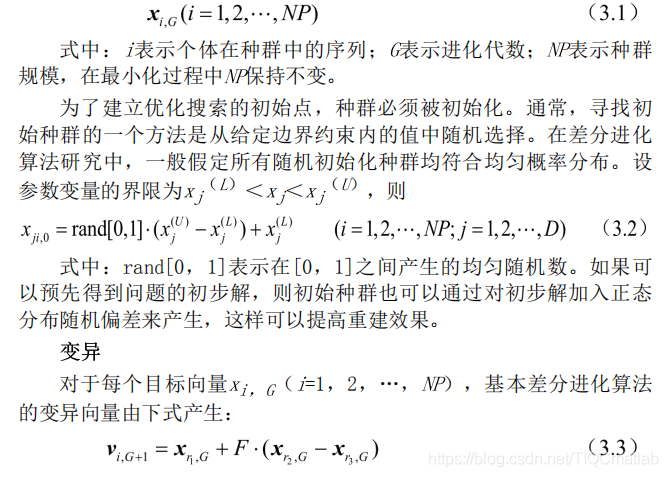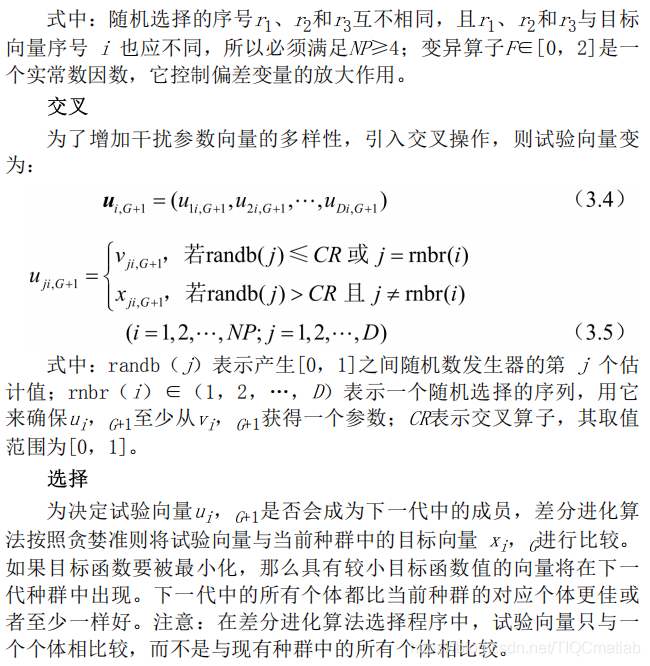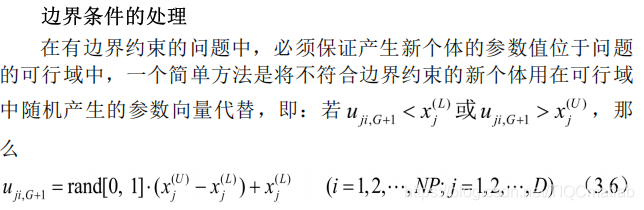另外一个方法是进行边界吸收处理，即将超过边界约束的个体值设置为临近的边界值。

3.2差分进化算法的其他形式 上面阐述的是最基本的差分进化算法操作程序，实际应用中还发展了差分进化算法的几个变形形式，用符号DE/x/y/z加以区分，其中：x限定当前被变异的向量是“随机的”或“最佳的”；y是所利用的差向量的个数；z指示交叉程序的操作方法。前面叙述的交叉操作表示为“bin”。利用这个表示方法， 基本差分进化算法策略可描述为DE/rand/1/bin。 还有其他形式[5，如：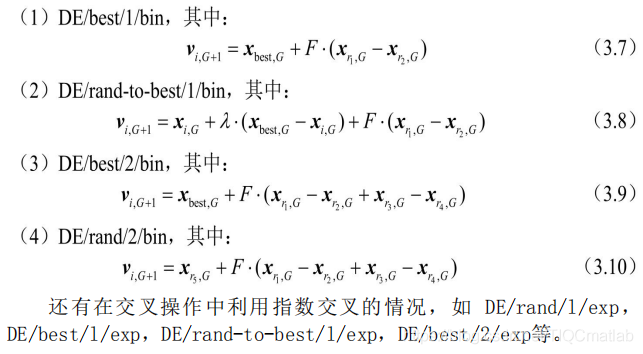3.3改进的差分进化算法 自适应差分进化算法 作为一种高效的并行优化算法，差分进化算法发展很快，出现了很多改进的差分进化算法。下面介绍一种具有自适应算子的差分进化算法.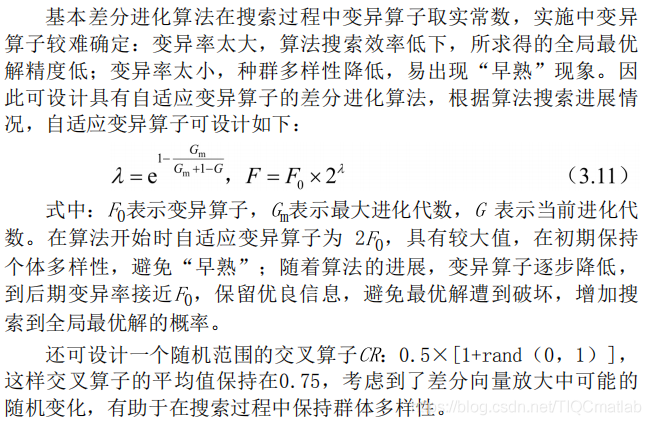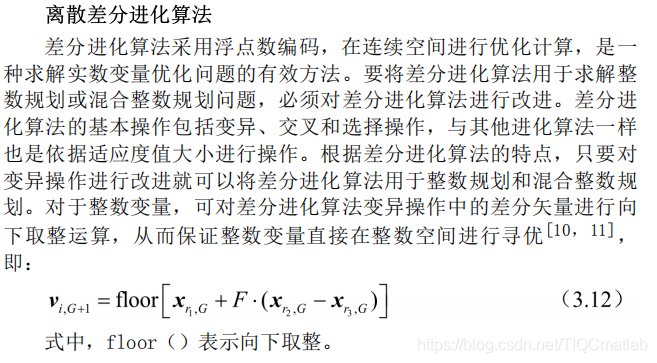4差分进化算法流程 差分进化算法采用实数编码、基于差分的简单变异操作和“一对一”的竞争生存策略，其具体步骤如下： (1)确定差分进化算法的控制参数和所要采用的具体策略。差分进化算法的控制参数包括：种群数量、变异算子、交叉算子、最大进化代数、终止条件等。 (2)随机产生初始种群，进化代数k=1。 (3)对初始种群进行评价，即计算初始种群中每个个体的目标函数值。 (4)判断是否达到终止条件或达到最大进化代数：若是，则进化终止，将此时的最佳个体作为解输出；否则，继续下一步操作。 (5)进行变异操作和交叉操作，对边界条件进行处理，得到临时种群。 (6)对临时种群进行评价，计算临时种群中每个个体的目标函数值。 (7)对临时种群中的个体和原种群中对应的个体，进行“一对-”的选择操作，得到新种群。 (8)进化代数k=k+1，转步骤(4)。 差分进化算法运算流程如图3.1所示。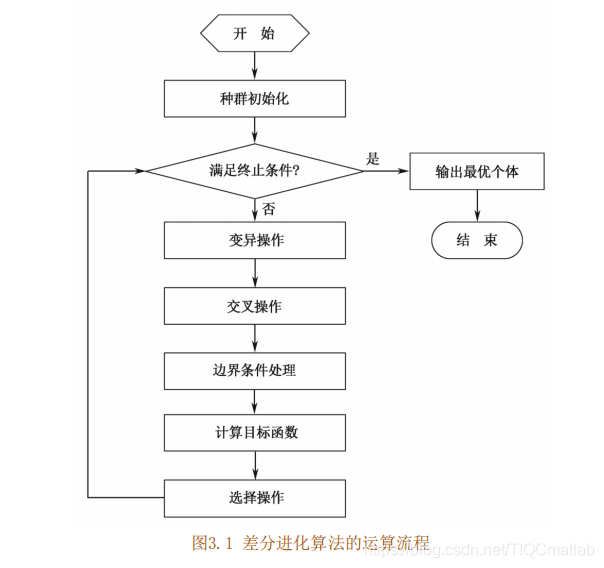5关键参数的说明 控制参数对一个全局优化算法的影响是很大的，差分进化算法的控制变量选择也有一些经验规则。

## 二、案例及完整源代码

1 案例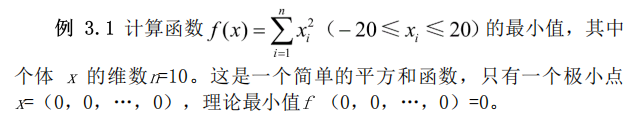2 完整代码 ```c %%%%%%%%%%%%%%%%%差分进化算法求函数极值%%%%%%%%%%%%%%%%%% %%%%%%%%%%%%%%%%%%%%%%%%%初始化%%%%%%%%%%%%%%%%%%%%%%%%% clear all; %清除所有变量 close all; %清图 clc; %清屏 NP=50; %个体数目 D=10; %变量的维数 G=200; %最大进化代数 F0=0.4; %初始变异算子 CR=0.1; %交叉算子 Xs=20; %上限 Xx=-20; %下限 yz=10^-6; %阈值 %%%%%%%%%%%%%%%%%%%%%%%%%赋初值%%%%%%%%%%%%%%%%%%%%%%%% x=zeros(D,NP); %初始种群 v=zeros(D,NP); %变异种群 u=zeros(D,NP); %选择种群 x=rand(D,NP)(Xs-Xx)+Xx; %赋初值 %%%%%%%%%%%%%%%%%%%%计算目标函数%%%%%%%%%%%%%%%%%%%% for m=1:NP Ob(m)=func1(x(:,m)); end trace(1)=min(Ob); %%%%%%%%%%%%%%%%%%%%%%%差分进化循环%%%%%%%%%%%%%%%%%%%%% for gen=1:G %%%%%%%%%%%%%%%%%%%%%%变异操作%%%%%%%%%%%%%%%%%%%%%% %%%%%%%%%%%%%%%%%%%自适应变异算子%%%%%%%%%%%%%%%%%%% lamda=exp(1-G/(G+1-gen)); F=F02^(lamda); %%%%%%%%%%%%%%%%%r1,r2,r3和m互不相同%%%%%%%%%%%%%%%% for m=1:NP r1=randi([1,NP],1,1); while (r1==m) r1=randi([1,NP],1,1); end r2=randi([1,NP],1,1); while (r2==m)|(r2==r1) r2=randi([1,NP],1,1); end r3=randi([1,NP],1,1); while (r3==m)|(r3==r1)|(r3==r2) r3=randi([1,NP],1,1); end v(:,m)=x(:,r1)+F(x(:,r2)-x(:,r3)); end %%%%%%%%%%%%%%%%%%%%%%交叉操作%%%%%%%%%%%%%%%%%%%%%%% r=randi([1,D],1,1); for n=1:D cr=rand(1); if (cr<=CR)|(n==r) u(n,:)=v(n,:); else u(n,:)=x(n,:); end end %%%%%%%%%%%%%%%%%%%边界条件的处理%%%%%%%%%%%%%%%%%%%%% for n=1:D for m=1:NP if (u(n,m)Xs) u(n,m)=rand(Xs-Xx)+Xx; end end end %%%%%%%%%%%%%%%%%%%%%%选择操作%%%%%%%%%%%%%%%%%%%%%%% for m=1:NP Ob1(m)=func1(u(:,m)); end for m=1:NP if Ob1(m)<Ob(m) x(:,m)=u(:,m); end end
for m=1:NP Ob(m)=func1(x(:,m)); end trace(gen+1)=min(Ob); if min(Ob(m))<yz break end end [SortOb,Index]=sort(Ob); x=x(:,Index); X=x(:,1); %最优变量
Y=min(Ob); %最优值
%%%%%%%%%%%%%%%%%%%%%%%%%画图%%%%%%%%%%%%%%%%%%%%%%%%%% figure plot(trace); xlabel('迭代次数') ylabel('目标函数值') title('适应度进化曲线')

```

```c %%%%%%%%%%%%%%%%%%%%%%%适应度函数%%%%%%%%%%%%%%%%%%%%%%%% function result=func1(x) summ=sum(x.^2); result=summ;```

## 三、解题过程及运行结果

1 解题过程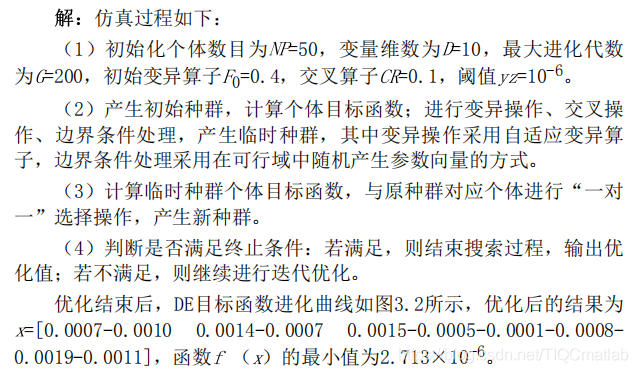2 运行结果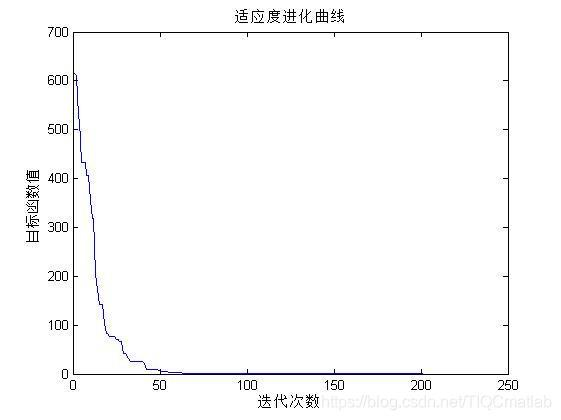## 四、matlab版本及参考文献

1 matlab版本 2014a

2 参考文献 《智能优化算法及其MATLAB实例（第2版）》包子阳 余继周 杨杉著 电子工业出版社